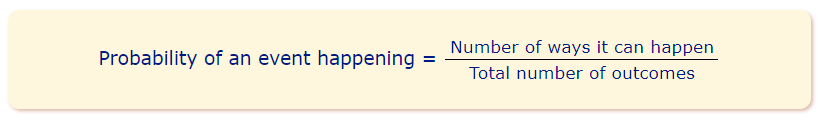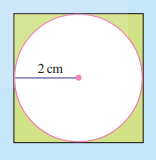# AP Board Class 9 Maths Chapter 14 Probability

The topic of probability is an important topic for Class 9 Maths students of Andhra board. They basically have to study the subject till Class 12. However, in Class 9, students will first be introduced to the topic in AP Board Class 9 Maths Chapter 14. In this unit, students will learn and understand, what probability means and go through some basic or fundamental concepts. The lessons on AP Board Class 9 Maths Chapter 14 Probability also include the following topics:

• Samples in probability
• Probability of events
• Probability problems

## What is Probability?

Generally, probability is defined as the likelihood or chance of an event that can occur. Probability plays a major role in many of our activities. However, there are circumstances and many events where we cannot accurately predict their outcome. Therefore, we use the idea of probability to determine what is or what could likely happen. A common example of probability would be tossing a coin and predicting the outcome either as heads or tails.

Probability is usually quantified as a number between 0 and 1 where,

• 0 indicates impossibility
• 1 indicates certainty
• The higher the probability of an event, the more likely it is that the event will occur.

### Probability FormulaStudents can check out some of the chapter questions along with their solutions to know more about the concept.

Question 1: A die has six faces numbered from 1 to 6. It is rolled and number on the top face is noted. When this is treated as a random trial.

1. What are the possible outcomes?
2. Are they equally likely? Why?
3. Find the probability of a composite number turning up on the top face.

Solution:

1. The number of possible outcomes is 6
2. Yes, they are equally likely because the probability of all the outcomes to occur individually is the same.
3. 4 and 6 are the only two composite number in the list. So, the probability of the composite number to turn up on the face can be calculated as follows:

= $$\frac{n(E)}{n(S)}=\frac{2}{6}$$

Question 2:

What is the probability that a randomly thrown dart that hits the square board in shaded region?Solution:

The radius of the circle inside the box is 2 cm.

Hence, the area of the circle can be calculated as

$$A=\pi r^2$$ $$A=3.14\times 2^2=12.56\, cm^2$$

The length of the square is 2cm + 2cm = 4 cm

Hence, the area of the square is $$16 cm^2$$

The area of the shaded region of the square board is

$$16-12.56=3.44\,cm^2$$

Hence, the probability can be calculated as follows:

$$\frac{3.44}{16}=0.215$$

The probability of a randomly thrown dart that hits the square board in shaded region is 0.215

Question 3: A coin is tossed 100 times and the following outcomes are recorded.
Head:45 times           Tails:55 times from the experiment
a) Compute the probability of each outcome.
b) Find the sum of probabilities of all outcomes.

Answer: Given that a coin is tossed 100 times, outcomes was head 45 times, while it showed tails 55 times.
(a) So, then, probability of head outcome is 100/45=0.45 and  the probability of tails outcome is 100/ 55=0/55
(b) The total outcomes 44+ 55, so that probability of the total outcome is 100/100

Know, more about Andhra Pradesh Board and access various study materials including syllabus, exam timetable, etc. at BYJU’s.